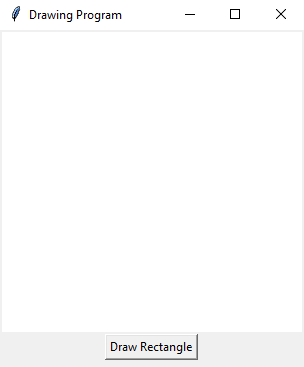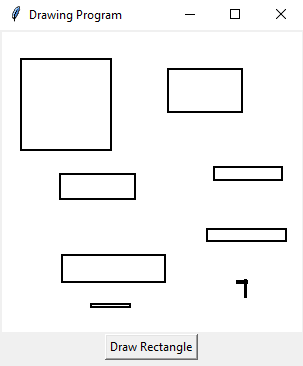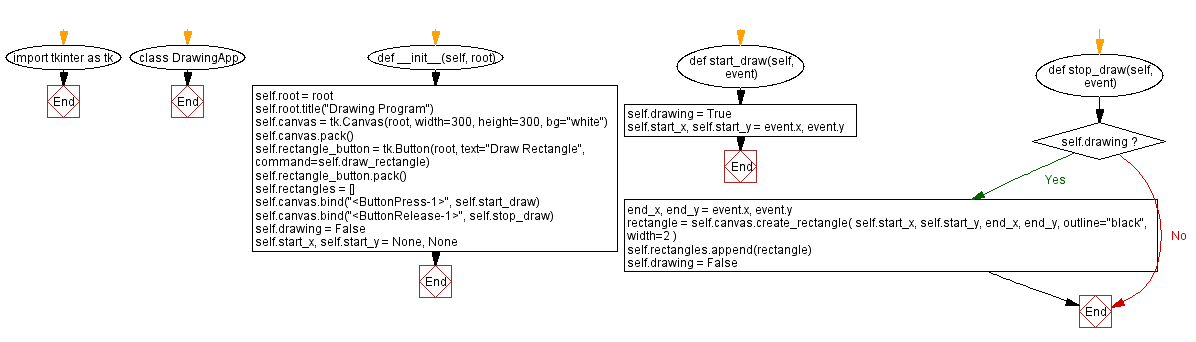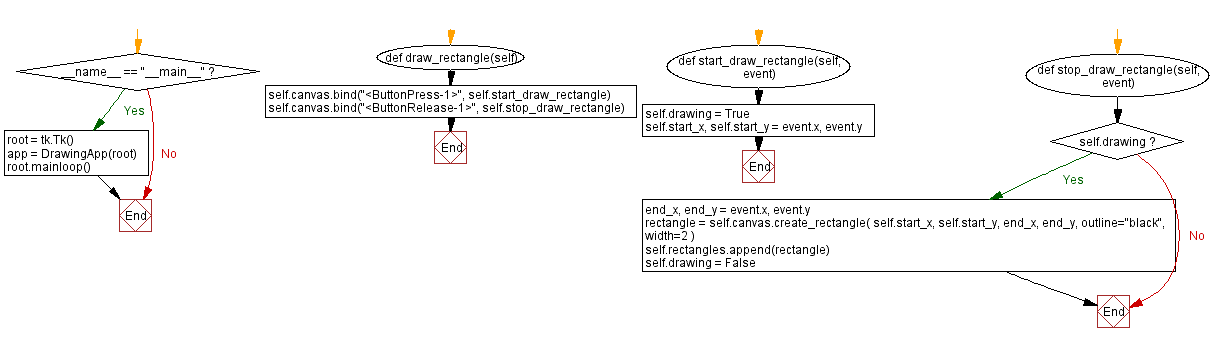﻿ Python Tkinter drawing program - Canvas shapes

# Building a drawing program with Python and Tkinter

## Python Tkinter Canvas and Graphics: Exercise-3 with Solution

Write a Python program that builds a simple drawing program with Tkinter and allows users to draw shapes (e.g., rectangles) on the Canvas.

Sample Solution:

Python Code:

``````import tkinter as tk

class DrawingApp:
def __init__(self, root):
self.root = root
self.root.title("Drawing Program")

self.canvas = tk.Canvas(root, width=300, height=300, bg="white")
self.canvas.pack()

self.rectangle_button = tk.Button(root, text="Draw Rectangle", command=self.draw_rectangle)
self.rectangle_button.pack()

self.rectangles = []

self.canvas.bind("<ButtonPress-1>", self.start_draw)
self.canvas.bind("<ButtonRelease-1>", self.stop_draw)

self.drawing = False
self.start_x, self.start_y = None, None

def start_draw(self, event):
self.drawing = True
self.start_x, self.start_y = event.x, event.y

def stop_draw(self, event):
if self.drawing:
end_x, end_y = event.x, event.y
rectangle = self.canvas.create_rectangle(
self.start_x, self.start_y, end_x, end_y, outline="black", width=2
)
self.rectangles.append(rectangle)
self.drawing = False

def draw_rectangle(self):
self.canvas.bind("<ButtonPress-1>", self.start_draw_rectangle)
self.canvas.bind("<ButtonRelease-1>", self.stop_draw_rectangle)

def start_draw_rectangle(self, event):
self.drawing = True
self.start_x, self.start_y = event.x, event.y

def stop_draw_rectangle(self, event):
if self.drawing:
end_x, end_y = event.x, event.y
rectangle = self.canvas.create_rectangle(
self.start_x, self.start_y, end_x, end_y, outline="black", width=2
)
self.rectangles.append(rectangle)
self.drawing = False

if __name__ == "__main__":
root = tk.Tk()
app = DrawingApp(root)
root.mainloop()
```
```

Explanation:

In the exercise above -

• Import the Tkinter library (tkinter).
• Create a DrawingApp class that manages the drawing program.
• The "start_draw()" and "stop_draw()" methods draw rectangles when the user clicks and releases the left mouse button.
• The "draw_rectangle()" method is called when the "Draw Rectangle" button is pressed. This allows the user to draw rectangles by clicking and dragging.
• Use the "<ButtonPress-1>" and "<ButtonRelease-1>" events to start and stop drawing actions.
• The drawn rectangles are stored in the self.rectangles list.
• The program sets up a basic Tkinter window with a canvas and a "button" to draw rectangles.
• The main event loop, root.mainloop(), starts the Tkinter application.

Output:

``````
``````

Flowchart:Python Code Editor:

What is the difficulty level of this exercise?

Test your Programming skills with w3resource's quiz.

﻿

## Python: Tips of the Day

Summing a sequence of numbers (calculating the sum of zero to ten with skips):

```>>> l = range(0,10,2)
>>> sum(l)
20
```

We are closing our Disqus commenting system for some maintenanace issues. You may write to us at reach[at]yahoo[dot]com or visit us at Facebook